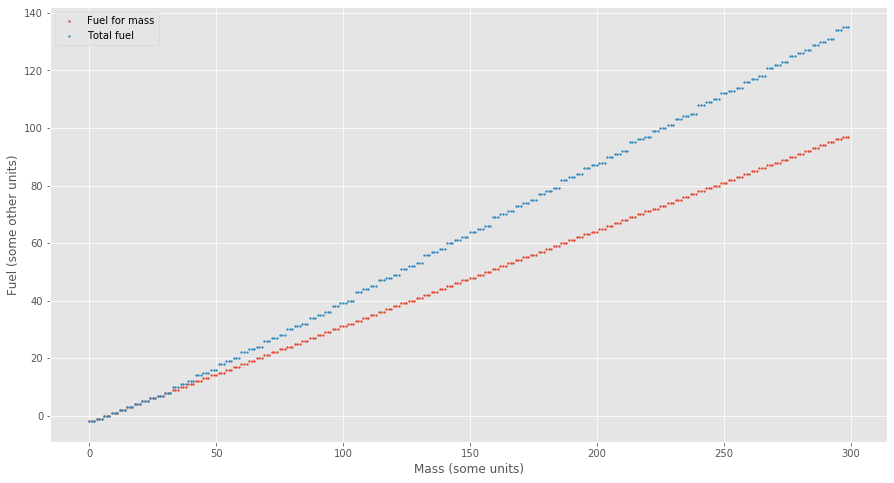# Advent of Code 2019 Day 1¶

In :
def required_fuel(mass: int) -> int:
return mass // 3 - 2

In :
for mass in [12, 14, 1969, 100756]:
print(f"{mass}: {required_fuel(mass)}")

12: 2
14: 2
1969: 654
100756: 33583

In :
input = []
with open("AoC/input1.txt") as input_file:
input = [int(x) for x in input_file.readlines()]

In :
sum(required_fuel(x) for x in input)

Out:
3267638
In :
def total_required_fuel(mass: int) -> int:
fuel = required_fuel(mass)
total_fuel = fuel
while fuel > 0: # I would love to use the walrus operator here
fuel = required_fuel(fuel)
if fuel > 0:
total_fuel += fuel

In :
for mass in [14, 1969, 100756]:
print(f"{mass}: {total_required_fuel(mass)}")

14: 2
1969: 966
100756: 50346

In :
sum(total_required_fuel(x) for x in input)

Out:
4898585
In :
limit = 300

import matplotlib.pyplot as plt
%matplotlib inline
plt.style.use('ggplot')

plt.figure(figsize=(15, 8))
plt.scatter(x=list(range(limit)), y=[required_fuel(x) for x in range(limit)], s=3, label="Fuel for mass")
plt.scatter(x=list(range(limit)), y=[total_required_fuel(x) for x in range(limit)], s=3, label="Total fuel")

plt.xlabel("Mass (some units)")
plt.ylabel("Fuel (some other units)")
plt.legend()

plt.show()